### 3.3. Effect on spectrum of radiation

Finally it is necessary to use the result for the frequency shift in a single scattering to calculate the form of the scattered spectrum of the CMBR. If every photon in the incident spectrum,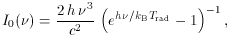(39)

is scattered once, then the resulting spectrum is given by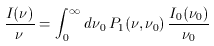(40)

where P1 (,0) is the probability that a scattering occurs from frequency0 to, and I() / his the spectrum in photon number terms. Since P1(,0) = P1 (s) /, where P1 (s) is the frequency shift function in (33), this can be rewritten as a convolution in s = ln (/0),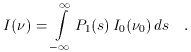(41)

The change in the radiation spectrum at frequencyis then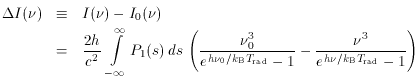(42)

where the normalization of P1 (s) has allowed the (trivial) integral over I0() to be included in (42) to give a form that is convenient for numerical calculation.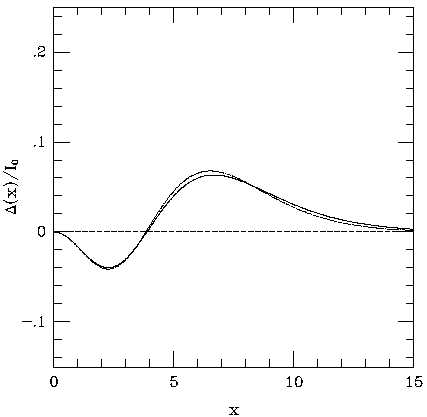Figure 7. The spectral deformation caused by inverse-Compton scattering of an incident Planck spectrum after a single scattering from a thermal population of electrons as a function of dimensionless frequency x = h/ kB Trad = 0.0176(/ GHz), with scaling I0 = 2 h / c2 [(kB Trad / h)]3. Left, for electrons at kB Te = 5.1 keV; right for electrons at kB Te = 15.3 keV. The result obtained from the Kompaneets kernel is shown as a dotted line. The shape of the distortion is independent of Te (and the amplitude is proportional to Te) for the Kompaneets kernel, but the relativistic expression leads to a more complicated form.

The integrations in (41) or (42) are performed using the P1 (s) function appropriate for the spectrum of the scattering electrons. The results are shown in Fig. 7 and 8 for two temperatures of the electron gas and for the power-law electron distribution. In these figures, x = h/ kBTrad is a dimensionless frequency. The functionsI (x) show broadly similar features for thermal or non-thermal electron distributions: a decrease in intensity at low frequency (where the mean upward shift of the photon frequencies caused by scattering causes the Rayleigh-Jeans part of the spectrum to shift to higher frequency, and hence to show an intensity decrease: see Fig. 1) and an increase in intensity in the Wien part of the spectrum. The detailed shapes of the spectra differ because of the different shapes of the scattering functions P1 (s) (Figs. 5 and 6).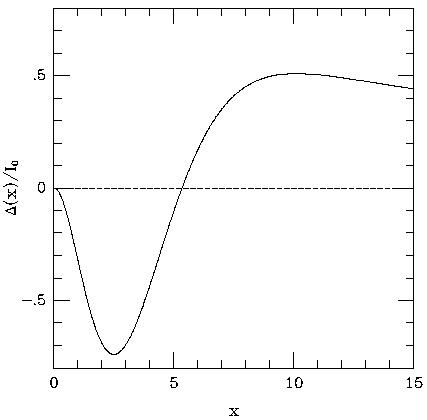Figure 8. The fractional spectral deformation caused by inverse-Compton scattering of an incident Planck spectrum by a single scattering from a power-law population of electrons with= 2.5 (equation 37). The spectral deformation has a similar shape to that seen in Fig. 7, but with a deeper minimum and more extended tail. This arises from the larger frequency shifts caused by the higher Lorentz factors of the electrons (see Fig. 6).

More generally, a photon entering the electron distribution may be scattered 0, 1, 2, or more times by encounters with the electrons. If the optical depth to scattering through the electron cloud ise, then the probability that a photon penetrates the cloud unscattered is e-e, the probability that it is once scattered ise e-e, and in general the probability of N scatterings is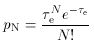(43)

and the full frequency redistribution function from scattering is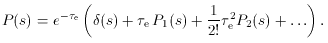(44)

The redistribution function Pn (s) after n scatterings is given by a repeated convolution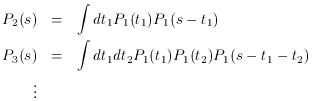(45)

but as pointed out by Taylor & Wright (1989), the expression for P (s) can be written in much simpler form using Fourier transforms, with P (s) obtained by the back transform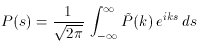(46)

of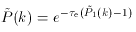(47)

where the Fourier transform of P1 (s) is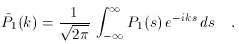(48)

The generalization of (41) for an arbitrary optical depth is then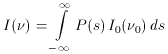(49)

but this full formalism will rarely be of interest, since in most situations the electron scattering medium is optically thin, withe << 1, so that the approximation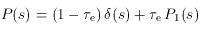(50)

will be sufficient (but see Molnar & Birkinshaw 1998b). The resulting intensity change has the form shown in Fig. 7 or 8, but with an amplitude reduced by a factore. This is given explicitly as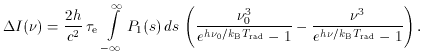(51)

and this form ofI() will be used extensively later. One important result is already clear from (51): the intensity change caused by the Sunyaev-Zel'dovich effect is redshift-independent, depending only on intrinsic properties of the scattering medium (through thee factor and P1 (s), and the Sunyaev-Zel'dovich effect is therefore a remarkably robust indicator of gas properties at a wide range of redshifts.